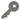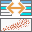# Generalized Linear RegressionAvailable in big data analytics.

The Generalized Linear Regression toolperforms Generalized Linear Regression (GLR) to generate predictions or to model a dependent variable in terms of its relationship to a set of explanatory variables. This tool can be used to fit Continuous (Gaussian), Count (Poisson), and Binary (Logistic) model types.

## Workflow diagram## Example

As an analyst for a large city, you have access to past 911 call records and demographic information. You need to answer the following questions: What variables effectively predict 911 call volume? Given future projections, what is the expected demand for emergency response resources?

## Usage notes

• This tool can be configured to perform one of two operational methods:
• Method 1—If only target (training) data is provided, the tool will fit a model to assess model performance. The tool then allows you to evaluate the performance of different models as you explore different explanatory variables and tool settings.
• Method 2—Once you have identified a good model and explanatory variables, configure the model to also provide join (prediction) data. When join data is configured, the tool will predict values for the dependent variable for features in your join (prediction) data based on the mapped explanatory variables.
• Use the Dependent variable parameter to select a field from the Target Input Layer (training data) representing the phenomena you are modeling. Use the Explanatory variables parameter to select one or more fields representing the explanatory variables from the Target Input Layer (training data). These fields must be numeric and have a range of values. Features that contain missing values in the dependent or explanatory variable will be excluded from the analysis. To modify null values, use the Calculate Field tool prior to updating values.
• The Generalized Linear Regression tool also produces output features and diagnostics. Output feature layers automatically have a rendering scheme applied to model residuals. A full explanation of each output is provided below.
• It is important to use the correct model type—Continuous (Gaussian), Count (Poisson), or Binary (Logistic)—for analysis to obtain accurate results of the regression analysis.
• Model summary results and diagnostics are written to the analytic logs as well as the output feature layer item details page. These diagnostics include a summary of the Generalized Linear Regression model and statistical summaries that are used to assess whether a model is a good fit for the data. The diagnostics reported depend on the model type chosen. The three options for Model type are as follows:
• Continuous (Gaussian)—Use if the dependent variable can take on a wide range of values such as temperature or total sales. Ideally, the dependent variable will be normally distributed.
• Count (Poisson)—Use if the dependent variable is discrete and represents the number of occurrences of an event such as a count of crimes. Count models can also be used if the dependent variable represents a rate and the denominator of the rate is a fixed value such as sales per month or number of people with cancer per 10,000 in the population. A Count (Poisson) model type assumes the mean and variance of the dependent variable are equal and the values of the dependent variable cannot be negative or contain decimals.
• Binary (Logistic)—Use if the dependent variable can take on one of two possible values such as success or failure, or presence or absence. The field containing the dependent variable must be numeric and contain only ones and zeros. There must be variation of the ones and zeros in the data.
• The Dependent variable and Explanatory variable(s) parameters should be numeric fields containing a range of values. This tool cannot solve when variables have the same values (for example, if all the values for a field are 9.0).
• Features with one or more null values or empty string values in prediction or explanatory fields will be excluded from the output. If necessary, modify values using the Calculate Field tool.
• Visually inspect the over- and under-predictions evident in the regression residuals to see whether they provide clues about potential missing variables from your regression model.
• Use the regression model that was created to make predictions for other features. Creating these predictions requires that each prediction feature (join dataset) has values for each of the explanatory variables specified. An explanatory variable mapping configuration is provided to map explanatory variable field names from the target (training) features and join (prediction) features. When matching the explanatory variable fields, the fields from the target (training data) and join (prediction data) features must be of the same type (for example, double fields must be matched with double fields).

## Parameters

ParameterDescriptionData type

Target Input Layer (training data)

The training features used to generate a model.

Features

Join Input Layer (prediction data)

(Optional)

The prediction features for which the dependent variable will be predicted based on the specified explanatory variables and model type.

This parameter is optional. If not specified, the Generalized Linear Regression tool will fit a model to assess model performance based on the training data.

Features

Model type

Specifies the model type to use. The model type chosen depends on the type of data in the dependent variable field. Model type options include the following:

• Continuous (Gaussian)—Choose if the dependent variable can take on a wide range of values such as temperature or total sales.
• Count (Poisson)—Choose if the dependent variable is discrete and represents the number of occurrences of an event such as a count of crimes or a rate and the denominator of the rate is a fixed value.
• Binary (Logistic)—Choose if the dependent variable can take on one of two possible values such as success or failure, or presence or absence.

String

Dependent variable

Specifies the field representing the phenomena you are modeling.

FieldName

Text to zero mapping

For theBinary (Logistic) model type, if a string field is specified for the Dependent variable, this parameter can be used to specify the string in the dependent variable to convert to zero.

String

Text to one mapping

For the Binary (Logistic) model type, if a string field is specified for the Dependent variable, this parameter can be used to specify the string in the dependent variable to convert to one.

String

Explanatory variable(s)

Field or fields from the target schema to represent independent explanatory variables in the regression model.

FieldNames

Explanatory variable mapping (prediction only)

Maps the selected explanatory variable field names in the target (training) schema to corresponding field names in join (predict) schema.

This parameter is optional. Explanatory variable mappings are only required to be specified if join (prediction) data is specified.

ExplanatoryVariableMappings

## Output layer

The Generalized Linear Regression tool produces a variety of outputs. A summary of the Generalized Linear Regression model and statistical summaries are available on the item details page of the output feature layer or in the analytic logs.

If implementing Method 1 of this tool to simply fit a model to assess performance, the training data will be the output, as well as messages and diagnostics available in the item details of the output feature layer in addition to results in the analytic logs.

If implementing Method 2 of this tool to fit a model and predict values, the prediction data will be the output with predicted values appended, as well as messages and diagnostics available in the item details of the output feature layer in addition to results in the analytic logs.

The diagnostics generated depend on the model type of the input features and are described below.

### Continuous (Gaussian)

#### Interpret messages and diagnostics

• AIC—This is a measure of model performance and can be used to compare regression models. Taking into account model complexity, the model with the lower AIC value provides a better fit to the observed data. AIC is not an absolute measure of goodness of fit but is useful for comparing models with different explanatory variables as long as they apply to the same dependent variable. If the AIC values for two models differ by more than 3, the model with the lower AIC value is considered more accurate.
• AICc—AICc applies a bias correction to AIC for small sample sizes. AICc will approach AIC as the number of features in the input increase. See AIC above.
• Multiple R-Squared—The R-Squared is a measure of goodness of fit. Its value varies from 0.0 to 1.0, with higher values being preferable. It may be interpreted as the proportion of dependent variable variance accounted for by the regression model. The denominator for the R-Squared computation is the sum of squared dependent variable values. Adding an extra explanatory variable to the model does not alter the denominator but does alter the numerator; this gives the impression of improvement in model fit that may not be real. See Adjusted R-Squared below.
• Adjusted R-Squared—Because of the problem described above for the R-Squared value, calculations for the adjusted R-Squared value normalize the numerator and denominator by their degrees of freedom. This has the effect of compensating for the number of variables in a model, and consequently, the Adjusted R-Squared value is almost always less than the R-Squared value. However, in making this adjustment, you lose the interpretation of the value as a proportion of the variance explained. In Geographically Weighted Regression (GWR), the effective number of degrees of freedom is a function of the neighborhood used, so the adjustment may be quite marked in comparison to a global model such as GLR. For this reason, AICc is preferred as a means of comparing models.

### Count (Poisson)

#### Interpret messages and diagnostics

• AIC—This is a measure of model performance and can be used to compare regression models. Taking into account model complexity, the model with the lower AIC value provides a better fit to the observed data. AIC is not an absolute measure of goodness of fit but is useful for comparing models with different explanatory variables as long as they apply to the same dependent variable. If the AIC values for two models differ by more than 3, the model with the lower AIC value is considered more accurate.
• AICc—AICc applies a bias correction to AIC for small sample sizes. AICc will approach AIC as the number of features in the input increase. See AIC above.

### Binary (Logistic)

#### Interpret messages and diagnostics

• AIC—This is a measure of model performance and can be used to compare regression models. Taking into account model complexity, the model with the lower AIC value provides a better fit to the observed data. AIC is not an absolute measure of goodness of fit but is useful for comparing models with different explanatory variables as long as they apply to the same dependent variable. If the AIC values for two models differ by more than 3, the model with the lower AIC value is considered more accurate.
• AICc—AICc applies a bias correction to AIC for small sample sizes. AICc will approach AIC as the number of features in the input increase. See AIC above.

## Considerations and limitations

The ArcGIS Velocity implementation of Generalized Linear Regression has the following limitations:

• It is a global regression model and does not take the spatial distribution of data into account.
• Analysis does not apply Moran's I test on the residuals.
• Points, lines, polygons, and tables are supported as target (training data) dataset geometry.
• You cannot classify values into multiple classes.